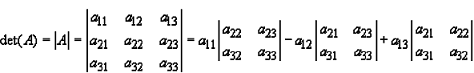## 3x3 matrix determinant calculator

 a = b= c = d = e= f = g = h= i =
 Determinant of Matrix(|A|)=

The 3x3 matrix determinant calculator to Find Determinant of a 3x3 Matrix

When you enter a 3x3 matrix click the "calculate" button. you will get the result.

### 3x3 Matrix Determinant FormulaThinkcalculator.com provides you helpful and handy calculator resources.